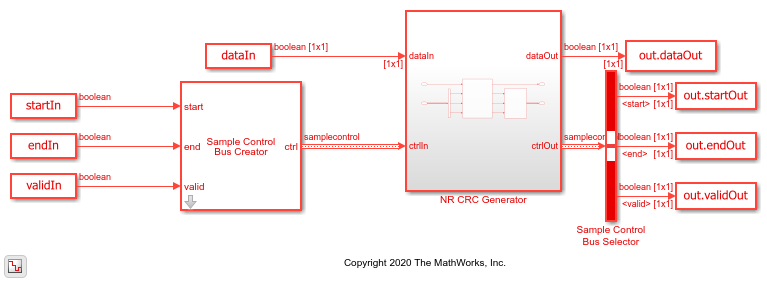# Encode Streaming Data Using General CRC Generator HDL Optimized Block for 5G NR Standard

This example shows how to use the General CRC Generator HDL Optimized block for encoding streaming data according to the 5G NR standard.

In this example, the output of this block is compared with the function `nrCRCEncode` (5G Toolbox). A cyclic redundancy check (CRC) is an error-detection code designed to detect errors in streaming data. A CRC generator calculates a short fixed-length binary sequence checksum and appends it with the data. A CRC detector performs a CRC on the data and compares the resulting checksum with the appended checksum. If the two checksums do not match, an error is detected. The CRC generator and detector are used in the 5G NR system to detect any errors in the transport blocks of control and uplink and downlink data channels. The 5G NR standard specifies six different cyclic generator polynomials: CRC6, CRC11, CRC16, CRC24A, CRC24B, and CRC24C. For more information about these polynomials, see TS 38.212 Section 5.1 [ 1 ].

### Generate Input Data for NR CRC Generator

Select a CRC polynomial specified in the 5G NR standard. Generate random input data of length `frameLen` and control signals that indicate the frame boundaries. The example model imports the MATLAB® workspace variables `dataIn`, `startIn`, `endIn`, `validIn`, `sampleTime`, and `simTime`.

```CRCType = 'CRC24A'; % Specify the CRCType as 'CRC6','CRC11','CRC16','CRC24A','CRC24B' or 'CRC24C' frameLen = 100; msg = randi([0 1],frameLen,1); [dataIn,ctrlIn] = whdlFramesToSamples(msg); dataIn = timeseries(logical(dataIn')); startIn = timeseries(logical(ctrlIn(:,1))); endIn = timeseries(logical(ctrlIn(:,2))); validIn = timeseries(logical(ctrlIn(:,3))); sampleTime = 1; simTime = length(ctrlIn(:,3)) + 100; ```

### Run NR CRC Generator Model

The `nrCRCGeneratorExampleInit.m` script configures the General CRC Generator HDL Optimized block by setting the parameters of the block based on the specified CRC generator polynomial, `CRCType`. This script also provides input to the reference function `nrCRCEncode` (5G Toolbox). The NR CRC Generator subsystem contains the General CRC Generator HDL Optimized block. Running the model imports the input signal variables from the workspace and returns the CRC-encoded output and control signals that indicate the frame boundaries. The model exports variables `encOut` and `ctrlOut` to the MATLAB® workspace.

```[poly,crcPolynomial,initState,finalXORValue] = nrCRCGeneratorExampleInit(CRCType); open_system('NRCRCGeneratorHDL'); encOut = sim('NRCRCGeneratorHDL'); ```### Verify NR CRC Generator Results

Convert the streaming data output of the NR CRC Generator subsystem to frames. Compare those frames with the output of the `nrCRCEncode` function.

```startIdx = find(encOut.startOut); endIdx = find(encOut.endOut); dataOut = encOut.dataOut; dataRef = nrCRCEncode(msg,poly); bitErr = sum(abs(dataRef - dataOut(startIdx:endIdx))); fprintf('CRC-encoded frame: Behavioral and HDL simulation differ by %d bits\n',bitErr); close_system('NRCRCGeneratorHDL'); ```
```CRC-encoded frame: Behavioral and HDL simulation differ by 0 bits ```

### References

1. 3GPP TS 38.212. NR ; Multiplexing and Channel Coding. 3rd Generation Partnership Project; Technical Specification Group Radio Access Network.